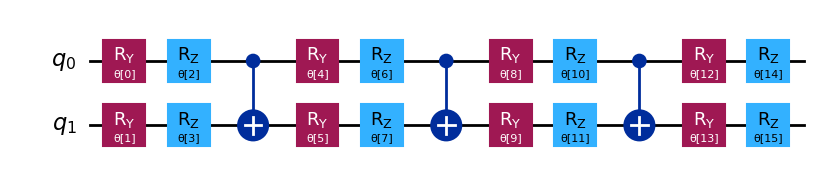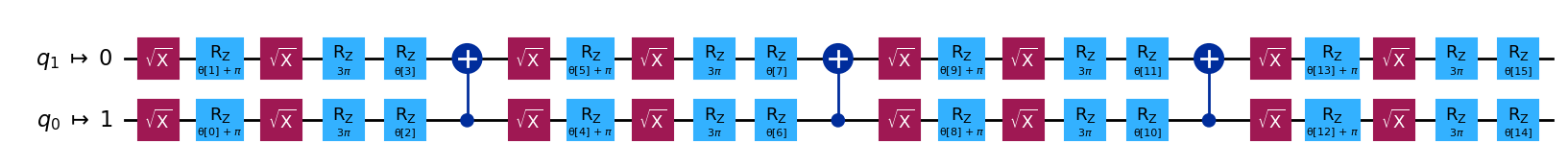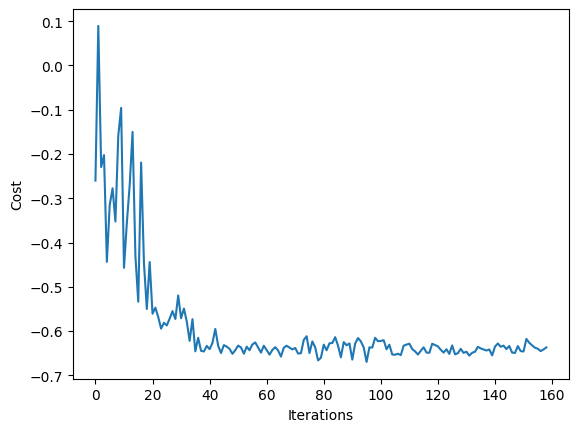Learning Home Catalog Composer Lab
Learning
Home Catalog Composer Lab
Variational quantum eigensolver
BackgroundSetupStep 1: Map classical inputs to a quantum problemStep 2: Optimize problem for quantum execution.Step 3: Execute using Qiskit Primitives.Creating a callback functionStep 4: Post-process, return result in classical format.

# Variational quantum eigensolver

Category

Workflow example

Topics

Scheduling

## Background

Variational quantum algorithms are promising candidate hybrid-algorithms for observing the utility of quantum computation on noisy near-term devices. Variational algorithms are characterized by the use of a classical optimization algorithm to iteratively update a parameterized trial solution, or "ansatz". Chief among these methods is the Variational Quantum Eigensolver (VQE) that aims to solve for the ground state of a given Hamiltonian represented as a linear combination of Pauli terms, with an ansatz circuit where the number of parameters to optimize over is polynomial in the number of qubits. Given that size of the full solution vector is exponential in the number of qubits, successful minimization using VQE requires, in general, additional problem specific information to define the structure of the ansatz circuit.

Executing a VQE algorithm requires the following 3 components:

1. Hamiltonian and ansatz (problem specification)
2. Qiskit Runtime estimator
3. Classical optimizer

Although the Hamiltonian and ansatz require domain specific knowledge to construct, these details are immaterial to the Runtime, and we can execute a wide class of VQE problems in the same manner.

## Setup

Here we import the tools needed for a VQE experiment. The primary imports can be grouped logically into three components that correspond to the three required elements.

Authenticate to run code cells
Reset Copy to clipboard

Output:

Authenticate to run code cells
Reset Copy to clipboard

Output:

'ibmq_manila'


## Step 1: Map classical inputs to a quantum problem

Here we define the problem instance for our VQE algorithm. Although the problem in question can come from a variety of domains, the form for execution through Qiskit Runtime is the same. Qiskit provides a convenience class for expressing Hamiltonians in Pauli form, and a collection of widely used ansatz circuits in the qiskit.circuit.library.

Here, our example Hamiltonian is derived from a quantum chemistry problem

Authenticate to run code cells
Reset Copy to clipboard

Output:

Our choice of ansatz is the EfficientSU2 that, by default, linearly entangles qubits, making it ideal for quantum hardware with limited connectivity.

Authenticate to run code cells
Reset Copy to clipboard

Output:From the previous figure we see that our ansatz circuit is defined by a vector of parameters, $\theta_{i}$, with the total number given by:

Authenticate to run code cells
Reset Copy to clipboard

Output:

16


## Step 2: Optimize problem for quantum execution.

We can schedule a series of qiskit.transpiler passes to optimize our circuit for a selected backend. This includes a few components:

• optimization_level: The lowest optimization level just does the bare minimum needed to get the circuit running on the device; it maps the circuit qubits to the device qubits and adds swap gates to allow all 2-qubit operations. The highest optimization level is much smarter and uses lots of tricks to reduce the overall gate count. Since multi-qubit gates have high error rates and qubits decohere over time, the shorter circuits should give better results.
• Dynamical Decoupling: We can apply a sequence of gates to idling qubits. This cancels out some unwanted interactions with the environment.
Authenticate to run code cells
Reset Copy to clipboard

Output:

Authenticate to run code cells
Reset Copy to clipboard

Output:We can also use apply_layout to transform our virtual observable to physical

Authenticate to run code cells
Reset Copy to clipboard

Output:

SparsePauliOp(['IIIZY', 'IIIIZ', 'IIIZZ', 'IIIXX'],
coeffs=[ 0.398 +0.j, -0.398 +0.j, -0.0113+0.j,  0.181 +0.j])


## Step 3: Execute using Qiskit Primitives.

Like many classical optimization problems, the solution to a VQE problem can be formulated as minimization of a scalar cost function. By definition, VQE looks to find the ground state solution to a Hamiltonian by optimizing the ansatz circuit parameters to minimize the expectation value (energy) of the Hamiltonian. With the Qiskit Runtime Estimator directly taking a Hamiltonian and parameterized ansatz, and returning the necessary energy, the cost function for a VQE instance is quite simple:

Authenticate to run code cells
Reset Copy to clipboard

Output:

Note that, in addition to the array of optimization parameters that must be the first argument, we use additional arguments to pass the terms needed in the cost function.

### Creating a callback function

Callback functions are a standard way for users to obtain additional information about the status of an iterative algorithm. The standard SciPy callback routine allows for returning only the interim vector at each iteration. However, it is possible to do much more than this. Here, we show how to use a mutable object, such as a dictionary, to store the current vector at each iteration, for example in case we need to restart the routine due to failure, and also return the current iteration number and average time per iteration.

Authenticate to run code cells
Reset Copy to clipboard

Output:

Authenticate to run code cells
Reset Copy to clipboard

Output:

We can now use a classical optimizer of our choice to minimize the cost function. Here, we use the COBYLA routine from SciPy through the minimize function. Note that when running on real quantum hardware, the choice of optimizer is important, as not all optimizers handle noisy cost function landscapes equally well.

To begin the routine, we specify a random initial set of parameters:

Authenticate to run code cells
Reset Copy to clipboard

Output:

Because we are sending a large number of jobs that we would like to execute together, we use a Session to execute all the generated circuits in one block. Here args is the standard SciPy way to supply the additional parameters needed by the cost function.

Authenticate to run code cells
Reset Copy to clipboard

Output:

Iters. done: 159 [Current cost: -0.6369410251499279]


At the terminus of this routine we have a result in the standard SciPy OptimizeResult format. From this we see that it took nfev number of cost function evaluations to obtain the solution vector of parameter angles (x) that, when plugged into the ansatz circuit, yield the approximate ground state solution we were looking for.

Authenticate to run code cells
Reset Copy to clipboard

Output:

 message: Optimization terminated successfully.
success: True
status: 1
fun: -0.6342900302279935
x: [ 5.653e+00  3.403e+00 ...  3.342e+00  6.535e+00]
nfev: 159
maxcv: 0.0


## Step 4: Post-process, return result in classical format.

If the procedure terminates correctly, then the prev_vector and iters values in our callback_dict dictionary should be equal to the solution vector and total number of function evaluations, respectively. This is easy to verify:

Authenticate to run code cells
Reset Copy to clipboard

Output:

True

Authenticate to run code cells
Reset Copy to clipboard

Output:

True


We can also now view the progress towards convergence as monitored by the cost history at each iteration:

Authenticate to run code cells
Reset Copy to clipboard

Output:Authenticate to run code cells
Reset Copy to clipboard

Output:

'0.14.1'

Authenticate to run code cells
Reset Copy to clipboard

Output:

'0.45.0'